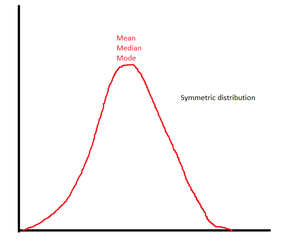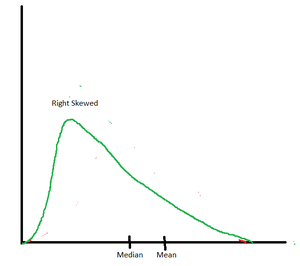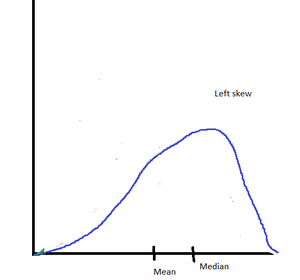Open in App
Not now

# Measures of Central Tendency

• Difficulty Level : Easy
• Last Updated : 06 Dec, 2022

Statistics is an important branch of mathematics that is widely used in a variety of traditional disciplines like economics, commerce, research, surveys, etc. In this present digital age, emerging technologies like data science and machine learning have boomed up. These technologies are also centered around statistics. After all, statistics is all about the collection, interpretation, and presentation of data. Basically, statistics provide insights into the data.

### Measures of Central Tendency

An essential statistical concept is the “measure of central tendency“. This measure is an important way to summarize the dataset with one representative value. This measure provides a rough picture of where data points are centered. The commonly used measures of central tendency are:

• Mean
• Median
• Mode

### Mean

“Average” value is termed as the mean of the dataset. It is very easy to calculate the mean.

Steps to calculate Mean:

• Step 1. Count the number of data values. Let it be n.
• Step 2. Add all the data values. Let the sum be s.
• Step 3. Mean = Sum of all data values (s)/Total number of data values(n)

### Median

The middle value of the sorted dataset is called the median.  Consider a dataset comprising ‘n’ elements.

Steps  to calculate median:

• Step 1. The dataset is arranged in either increasing or decreasing order.
• Step 2. If the data set has an odd number of data values (n=odd), then the middlemost value of the sorted dataset is computed as the median. In other words, the data at (n + 1)/2 place is the median of the dataset.
• Step 3. If the dataset has an even number of data values (n = even), the average of two middle values is computed as the median. i.e. mean of (n/2) and {(n/2) + 1}th is the median of the dataset.

### Mode

The most frequently occurring value in the dataset is called mode.

Steps to calculate mode:

• Step 1. Use tally marks to identify how many times each data value occurs in the dataset.
• Step 2. The data value with maximum tally is the mode of the dataset.

### Examples

Example 1. Consider the weight (in kg) of 5 children as 36, 40, 32, 42, 30. Let’s compute mean, median, and mode:

Solution:

• Mean = (36 + 40 + 32 + 42 + 30)/5 = 180/5 = 36kg
• Median: Arrange the data in ascending order: 30, 32, 36, 40, 42 The middle value is 36. So, median = 36kg.
• Mode: 36 kg occurs most number of times, so mode = 36 kg

In this example, we saw that mean, median and mode are same.

Example 2. Consider the ages of five employees as 30, 30, 32, 38, 60 years. Calculate the measures of central tendency.

Solution:

• Mean = (30 + 30 + 32 + 38 + 60)/5 = 190/5 = 38 years
• Median: Arrange the data in ascending order: 30, 30, 32, 38, 60. The middlemost value is 32. So, median = 32 years
• Mode: 30 years occurs most number of ties, so mode = 30 years

In this example, we saw that mean, median and mode have different values.

Example 3. Five students A, B, C, D, E appeared in a test and scored 80, 95, 90, 85, and 100 marks respectively. Find the mean?

Solution:

Total number of students = 5

Sum of marks = 80 + 95 + 90 + 85 +100 = 450

Mean = Sum of marks/total number of students

= 450/5 = 90 marks

Example 4. A batsman scores an average of 48 runs in six matches. If his score in five matches is 51, 45,46, 44, and 49. Find his score in the sixth match?

Solution:

Total number of matches = 6

Assume his score in sixth match = x runs

Average = 48 runs

So, (51 + 45 + 46 + 44 + 49 + x)/6 = 48

So, 235 + x = 48 x 6 = 288 = 235 + x = 288

x = 288 – 235 = 53

He scores 53 runs in sixth match.

Example 5. The average of five consecutive odd numbers is 15. Find the numbers?

Solution:

Assume the smallest odd number be x.

So, the other numbers are x + 2, x + 4, x + 6, x + 8

Given that the average = 15.

So, (x + x + 2 + x + 4 + x + 6 + x + 8)/5 = 15

= 5x + 20 = 75

= 5x = 55

x = 55/5 = 11

So, the numbers are 11, 13, 15, 17, 19

Example 6. A teacher reported a mean of 35 marks in a class of 20 students. Later she realized that marks of a student were actually 45, but by mistake, she had written as 25. Find the correct mean marks of the class.

Solution:

Mean = 35

Number of students = 20

So, total sum of marks = 35 × 20 = 700

Corrected sum of marks = 700 – 25 + 45 = 720

So, average = 720/20 = 36

Correct mean = 36 marks

### Distributions and Mean

Mean is highly impacted by the extreme values in the dataset. If the dataset is symmetric, the mean value is located exactly at the center. However, in skewed distributions, the mean value is pulled away from the center.

Case 1: Symmetric distribution

Consider a symmetric distribution. Assume the monthly salary of employees in an organization as 30k, 40k, 35k, 32k, 38k rupees.

Mean = (30 + 40 + 35 + 32 + 38)/5 = 175/5 = 35k rupees

Median: Sort the data in ascending order. 30k, 32k, 35k, 38k, 40k. Since the middlemost value in the sorted dataset is 35k. We can conclude that median salary = 35k rupees. No clear mode as all the data value occurs the same number of times.Mean = Median = mode in symmetric distribution

Case 2: Skewed distribution

In skewed distribution where one value is exceptionally different from other values, the mean value changes drastically.Mean > median in right skew distributionMean < median in left-skewed distribution

Let us assume a scenario where an employee is promoted, and he gets an awesome hike in salary. Assume that his salary changes from 38k per month to 85k per month. This is a case of right skew as the data value has been shifted towards the right. According to the figure, we expect that mean should be more than the median.

Let us compute the new values of mean & median

New dataset has values 30, 40, 35, 32, 88

Mean = (30 + 40 + 35 + 32 + 88) = 225/5 = 45k rupees

Median:

Sort the data in ascending order.

30k, 32k, 35k, 40k,88k

Since the middlemost value in the sorted dataset is 35k, we can conclude that median salary = 35k rupees. Thus, we saw that the mean value changed, but the median value is still 35k rupees. It is evident that the mean value is extremely sensitive to changes in data. However, the median is relatively stable.

### The Best Measure of Central Tendency

• Mean is the preferred measure of central tendency when data is normally distributed.
• Median is the best measure of central tendency when data is skewed.
• While dealing with nominal variables, the mode is the best measure of central tendency.

### Conclusion

• Mean, median, and mode are the most important measures of central tendency. The complete dataset may be represented by these values.
• It is not necessary for mean, median, and mode to have the same values.
• Mean is sensitive to extreme data values.
• It is not wise to take the mean of skewed distribution as the true representative of the dataset.
• Median is a better way to understand skewed distribution.
• Mean and median can not be zero unless all data values are zero. However, it is possible that there is no mode in the dataset.
My Personal Notes arrow_drop_up
Related Articles